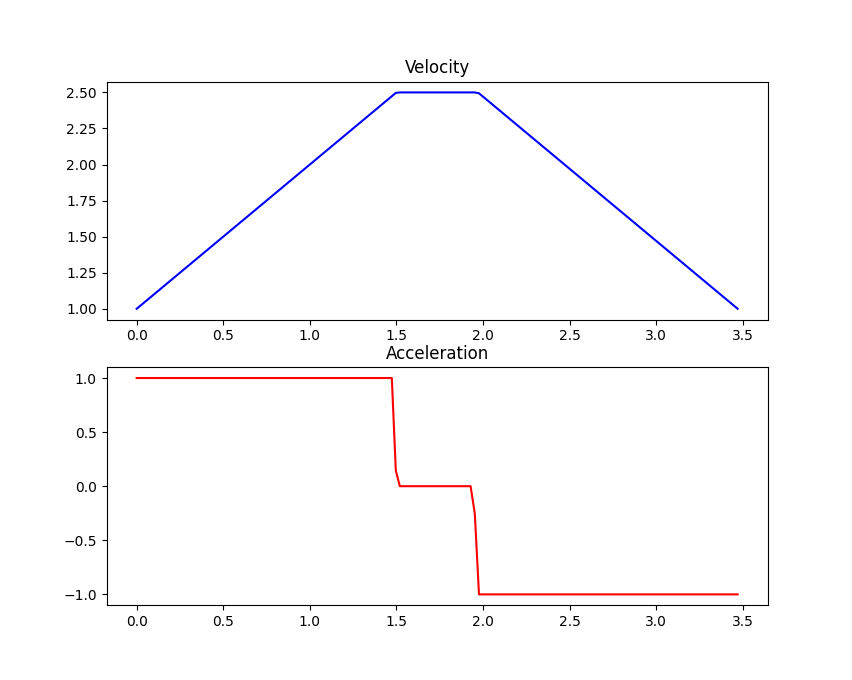# Constraints¶The robot’s Constraints provide the maximum allowable dynamics for the generated paths. Careful measurement and configuration of these parameters ensures that the path will not expect the robot to move more quickly than it actually can. Resolving such discrepancies in the generated path and reality is an important first step in ensuring that the robot performs reliably.

## Maximum Velocity¶

The simplest constraint for the robot’s motion is its Maximum Velocity. This value can be found by one of two methods: calculation and measurement. There are a lot of options for measuring the maximum velocity; we’ll focus on the calculation option here.

Calculating the theoretical maximum velocity can be done easily without using the actual robot. So long as you know the wheel diameter and motor velocity for the drivetrain you can calculate the robot’s maximum velocity.

Let’s say we have 4 inch wheels and 200rpm motors on the robot’s drive. We’ll first convert these values into the right units:

4 inches * 0.0254 inches/meter = 0.1016 meters

200 rpm / 60 seconds/minute = 3.333 revolutions per second


We then find the circumference of the wheels and multiply by the rate of rotation of the motor to get the velocity.

Circumference = PI * Diameter = 3.14 * 0.1016 = 0.319 meters
Velocity = Circumference * Rotation Rate = 0.319 * 3.333 = 1.063 meters per second


## Maximum Acceleration¶

Let’s assume that we’re using the same robot as above with the 4 inch wheels and 200 rpm motors. If we’re using the VEX V5 Smart Motors <https://www.vexrobotics.com/276-4840.html#additional> on the drive then our 200 rpm motors will have a stall torque of 1.05 Nm. We won’t be able to hit that value while keeping the robot moving and we don’t want to push the motors that hard all the time. Let’s set our maximum torque at 0.5 Nm for the robot’s movements to keep the current down and the motors happy.

We can use the physics equations for torque and force to find the maximum acceleration for our robot. First, let’s find the maximum force that the robot can deliver.

Torque = wheel radius * force
0.5 Nm = (0.1016 meters / 2) * force
Force = 9.843 N


We can then use Newton’s second law and the mass of our fictional robot to find the maximum acceleration. It is important to remember that we are looking for the sum of the forces so we will account for each motor on the drive. Let’s give our fictional robot 4 drive motors for a total of 9.843 * 4 = 39.372 Newtons.

Sum of Force = mass * acceleration
39.372 = 10 Kg * acceleration
acceleration = 3.937 meters per second per second


## Maximum Jerk¶

The calculations for maximum jerk are not nearly as convenient as the above calculations for velocity and acceleration. The easiest option for this parameter is to set it to an arbitrary value that’s a bit larger than your acceleration, like twice as large. This can be a good fiddle-factor to get the robot’s movement to be smoother or more aggressive than default.